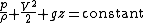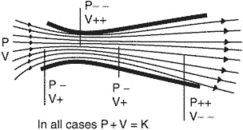Bernoulli's theorem

(redirected from Bernoullis theorem)
Also found in: Dictionary.

Bernoulli's theorem

An idealized algebraic relation between pressure, velocity, and elevation for flow of an inviscid fluid. Its most commonly used form is for steady flow of an incompressible fluid, and is given by the equation below,where p is pressure, &rgr; is fluid density (assumed constant), V is flow velocity, g is the acceleration of gravity, and z is the elevation of the fluid particle. The relation applies along any particular streamline of the flow. The constant may vary across streamlines unless it can be further shown that the fluid has zero local angular velocity.

The above equation may be extended to steady compressible flow (where changes in &rgr; are important) by adding the internal energy per unit mass, e, to the left-hand side. See Compressible flow

The equation is limited to inviscid flows with no heat transfer, shaft work, or shear work. Although no real fluid truly meets these conditions, the relation is quite accurate in free-flow or “core” regions away from solid boundaries or wavy interfaces, especially for gases and light liquids. Thus Bernoulli's theorem is commonly used to analyze flow outside the boundary layer, flow in supersonic nozzles, flow over airfoils, and many other practical problems. See Aerodynamics, Boundary-layer flow

McGraw-Hill Concise Encyclopedia of Physics. © 2002 by The McGraw-Hill Companies, Inc.
The following article is from The Great Soviet Encyclopedia (1979). It might be outdated or ideologically biased.

Bernoulli’s Theorem

one of the major theorems of the theory of probability; it is the simplest case of the so-called law of large numbers. Bernoulli’s theorem was first published in Jakob Bernoulli’s treatise Ars Conjectandi, published in 1713. The first proofs of Bernoulli’s theorem required complex mathematical methods, and only in the mid-19th century did P. L. Chebyshev find an unusually elegant and short proof of it. The exact formulation of Bernoulli’s theorem is the following: if for each of n independent trials the probability of a certain event is equal to p, then the probability that the frequency m\n of occurrence of the event satisfies the inequality ǀm/n - pǀ< ε(ε is an arbitrarily small positive number) becomes as close to 1 as desired for a sufficiently large number n of trials. The following simple quantitative estimate of this probability results from Chebyshev’s proof:

P{ǀm/n - p \ <ε} > 1 - p(1 - p)/nε2

V. I. BITIUTSKOV